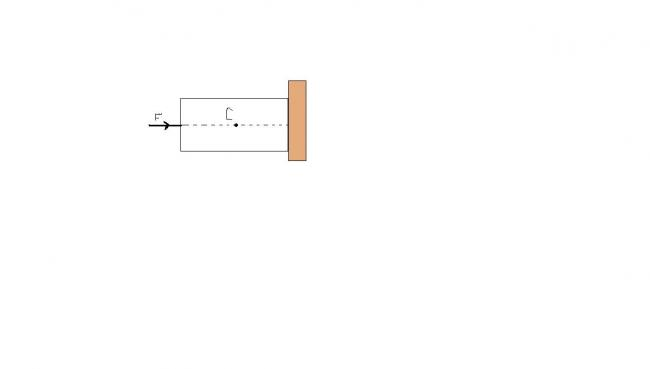# solve karo

A cube of mass 2kg is held stationary against a rough wall by a force F=40N pass through centre C.Find perpendicular distance of normal reaction between wall and cube from point C.Side of the cube is 20cm.•eureka123 ·

have u drawn fbd????

if yes then answer will be in front of u...

•nix_13 12 ·

hmmmmm.........i did draw FBD............but still didnt get.............

•Physics Wallah ·

JUST SEE THAT friction=2g=20N
NORMAL REACTION=40N
HENCE,ABOVE WE KNOW THAT SYSTEM IS IN REST
SO AT MIDPOINT C Total friction distance from c is 10 and the normal reaction assume x then 20*10=N*x solving this we get x=5cm

•